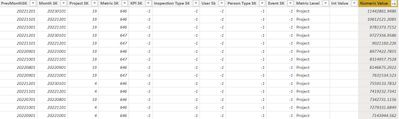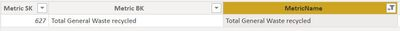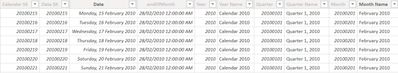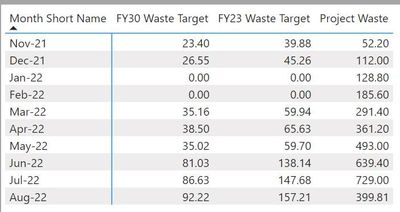cancel
Showing results for
Did you mean:Helper I

## Sum for current month

Hi All,

I need to sum a numeric value for the current month, each month. Data model has a realtionship between Metric[Month SK] and Calendar[Date SK].

data table: Metriclookup label: Metric NameDate Table:CalendarLine Chart as table with expected value 399.81Measure in line chart:

CALCULATE (SUM('Metric'[Numeric Value])

,'Metric Name'[MetricName] =  "Total General Waste recycled"

+

CALCULATE (SUM('Metric'[Numeric Value])

,'Metric Name'[MetricName] =  "Total General Waste sent to landfill"

)

Current Month Measure returns blank:

# Total Waste Current Month =

VAR CurrentMonth = MONTH(TODAY())

VAR TotalWasteMTD = CALCULATE (

SUM('Metric'[Numeric Value]),'Metric Name'[MetricName] =  "Total General Waste recycled",

'Calendar'[Month] = CurrentMonth

)

+

CALCULATE (SUM('Metric'[Numeric Value]),'Metric Name'[MetricName] =  "Total General Waste sent to landfill",

'Calendar'[Month] = CurrentMonth

)

RETURN

TotalwasteMTD

This should be simple but I'm not sure what's wrong.

Thanks

1 ACCEPTED SOLUTIONSuper User

@ringovski , Try like

calculate( SUM('Metric'[Numeric Value]),filter('Metric Name' ,'Metric Name'[MetricName] = "Total General Waste recycled"),Filter('Calendar' ,eomonth('Calendar'[Date],0) = eomonth(Today(),0)))
+calculate( SUM('Metric'[Numeric Value]),filter('Metric Name' ,'Metric Name'[MetricName] = "Total General Waste sent to landfill"),Filter('Calendar' ,eomonth('Calendar'[Date],0) = eomonth(Today(),0)))

If some date is selected and you want to ignore

calculate( SUM('Metric'[Numeric Value]),filter('Metric Name' ,'Metric Name'[MetricName] = "Total General Waste recycled"),Filter(all('Calendar' ),eomonth('Calendar'[Date],0) = eomonth(Today(),0)))
+calculate( SUM('Metric'[Numeric Value]),filter('Metric Name' ,'Metric Name'[MetricName] = "Total General Waste sent to landfill"),Filter(all('Calendar' ),eomonth('Calendar'[Date],0) = eomonth(Today(),0)))

!! Microsoft Fabric !!
Microsoft Power BI Learning Resources, 2023 !!
Learn Power BI - Full Course with Dec-2022, with Window, Index, Offset, 100+ Topics !!
Did I answer your question? Mark my post as a solution! Appreciate your Kudos !! Proud to be a Super User! !!Super User

@ringovski , Try like

calculate( SUM('Metric'[Numeric Value]),filter('Metric Name' ,'Metric Name'[MetricName] = "Total General Waste recycled"),Filter('Calendar' ,eomonth('Calendar'[Date],0) = eomonth(Today(),0)))
+calculate( SUM('Metric'[Numeric Value]),filter('Metric Name' ,'Metric Name'[MetricName] = "Total General Waste sent to landfill"),Filter('Calendar' ,eomonth('Calendar'[Date],0) = eomonth(Today(),0)))

If some date is selected and you want to ignore

calculate( SUM('Metric'[Numeric Value]),filter('Metric Name' ,'Metric Name'[MetricName] = "Total General Waste recycled"),Filter(all('Calendar' ),eomonth('Calendar'[Date],0) = eomonth(Today(),0)))
+calculate( SUM('Metric'[Numeric Value]),filter('Metric Name' ,'Metric Name'[MetricName] = "Total General Waste sent to landfill"),Filter(all('Calendar' ),eomonth('Calendar'[Date],0) = eomonth(Today(),0)))

!! Microsoft Fabric !!
Microsoft Power BI Learning Resources, 2023 !!
Learn Power BI - Full Course with Dec-2022, with Window, Index, Offset, 100+ Topics !!
Did I answer your question? Mark my post as a solution! Appreciate your Kudos !! Proud to be a Super User! !!